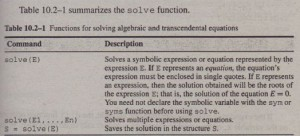Algebraic and Transcendental Equations
The Symbolic Math toolbox can solve algebraic and transcendental equations, well as systems of such equations. A transcendentaL equation is one that contain one or more transcendental functions, such as sin x, e”, or log x. The appropriate function to solve such equations is the sol ve function. The function solve (E) solves a symbolic expression or equation represented by the expression E. If E represents an equation, the equation’s expressi
must be enclosed in single quotes. If E represents an expression, then the soiuti obtained will be the roots of the expression E; that is, the solution ofthe equ . E =O.Multiple expressions or equations can be solved by separatin.&-themwi a comma, as sol ve (E1, E2, … , En). Note that you need not declare symbolic variable with the sym or syms function before using solve. There are three ways o use the solve function.For example, to solve
equation x + 5 = 0, one way is »eql = ‘x+S=O’; »solve(eql)

= -5 second way is • -5 third way is -5 can store the result in a named variable as follows: -5 To solve the equation e2x + 3e-t = 54, the session is _olve(‘exp(2*x)+3*exp(x)=54’) log(-9)) . log(6)) that log (6) is In(6) = 1.7918, whereas log (-9),which is In(-9), is a lex number. To see this, in MATLAB type log (-9) to obtain 2.1972 +
16;. So we obtained two solutions, instead of one, and now we must decide ••• er both are meaningful. The answer depends on the application that prodteoriginaJ~ n. If the applicationrequires areal number for a solution,
e should choose log (6) as the answer. following se iOM provide iOJDe more examples of the use of these
2 = ‘yA2+3*y+2=O’~
ve(eq2) = (-2J [-1J 3 = ‘xA2+9*yA4=O’;
ve(eq31 , ate that K is presumed to be the unknown variable
= { 3*i*y”‘2]

When more than one variable occurs in the expression, MATLAB assumes that the variable closest to x in the alphabet is the variable to be found. You can specify the solution variable using the syntax sol ve (E, ,v ‘ ), where v is the solution variable. For example, »solve(‘bA2+8*c+2*b=O’) %solves for c because it is closer to x
ans =
-1/8*bA2-1/4*b
»solve(‘bA2+8*c+2*b=O’, ‘0’) % solves for b
ans
-1+(1-8*c)A(1/2)]
-1-(1-8*c)A(1/2)]
Thus the solution of b2 + 8c + 2b = 0 for cis c = _(b2 + 2b)/8. The solution
for b is b = -1 ± .JI=8C.
You can save the solutions as vectors by using the form [x, y] ‘= so 1ve (eql, eq2). Note the difference in the output formats in the following example:
»eq4 = ‘6*x+2*y=14’j
»eq5 = ‘3*x+7*y=31’j
»solve(eq4,eq5)
ans
x: [lxl sym]
y: [lxl sym]
»x ans.x
x =
1
»y = ans.y =
4
»[i, y) = solve(eq4,eq5)
x
1
y
SOLUTION
STRUCTURE
4
You can save the solution in a structure with named fields (see Chapter 2, Section 2.7 for a discussion of structures and fields). The individual solutions are saved in the fields. For example, continue the preceding session as follows:
»8 = solve(eq4,eq5)
s
x: [lxl sym]
y: [lxl sym]

T10.2-1 Use MATLAB to solve the equation Jf’=X’2 = x.
T10.2-2 Use MATLAB to solve the equation set x + 6y = a, 2x – 3y = 9 for x and y in terms of the parameter a.
(Answer: x = (a + 18)/5, y = (2a – 9)/15.)

T10.2-3 Find the y coordinates of the intersection points in Example io.z-r.
b =,./3. .